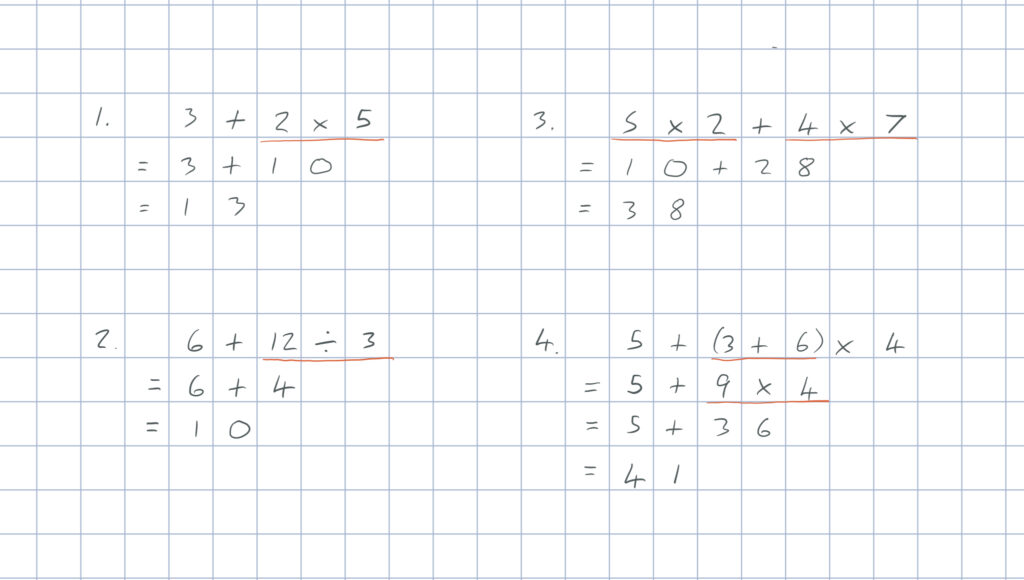BODMAS is an acronym for the order in which we complete operations. This acronym comes in various forms and typically stands for Brackets, Order (power), Divide, Multiply, Add and Subtract. We can divide or multiply in either order and we can add or subtract in either order, but addition and subtraction must follow multiplication and division, which must follow expanding brackets and powers.